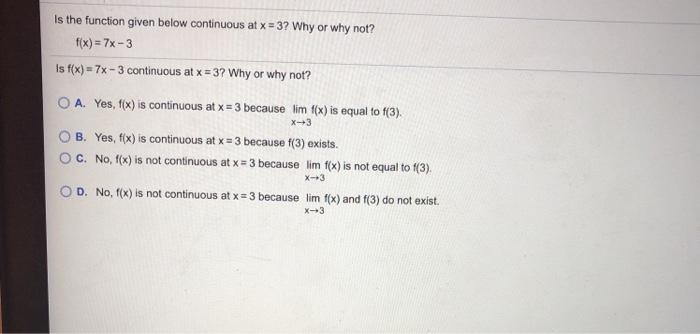### Create an Account

Already have account?

### Forgot Your Password ?

Home / Questions / Is the function given below continuous at x = 3? Why or why not? f(x) = 7x-3 Is f(x) = 7x ...

# Is the function given below continuous at x = 3? Why or why not? f(x) = 7x-3 Is f(x) = 7x - 3 continuous at x = 3? Why or why not? O A. Yes, f(x) is continuous at x = 3 because lim f(x) is equal to f(

Is the function given below continuous at x = 3? Why or why not? f(x) = 7x-3 Is f(x) = 7x - 3 continuous at x = 3? Why or why not? O A. Yes, f(x) is continuous at x = 3 because lim f(x) is equal to f(3). X-3 B. Yes, f(x) is continuous at x = 3 because f(3) exists. OC. No, f(x) is not continuous at x = 3 because lim f(x) is not equal to f(3). X-3 OD. No, f(x) is not continuous at x = 3 because lim f(x) and f(3) do not exist. X-3Apr 19 2021 View more View Less

#### Answer (Solved)Subscribe To Get Solution